# 12-240/Classnotes for Thursday November 8

(diff) ← Older revision | Latest revision (diff) | Newer revision → (diff)

## Riddle Along

Four cars drive in the Sahara desert at constant speeds and with constant directions. A meets B, C, D; B meets C, D. Do C and D meet?

## Goals

1. compute Rank T over A
2. Compute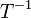$T^{-1}$ over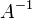$A^{-1}$
3. Solve systems of linear equations

## Theorems

1. Given V' -> V -> W -> W' (where the linear transformations are Q, T, P respectively)
such that P and Q are invertible (i.e. Q is surjective and P is injective) then rank T = rank PTQ

2. if T: V -> W, V with basis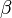$\beta$ and W with basis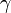$\gamma$ rank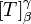$[T]_\beta^\gamma$ = rank T

3. if P and Q are invertible matrices, A is some other matrix, rank A = rank PAQ

## Definitions

if A =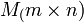$M_(m \times n)$, then it is linear transformation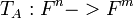$T_A : F^n -> F^m$